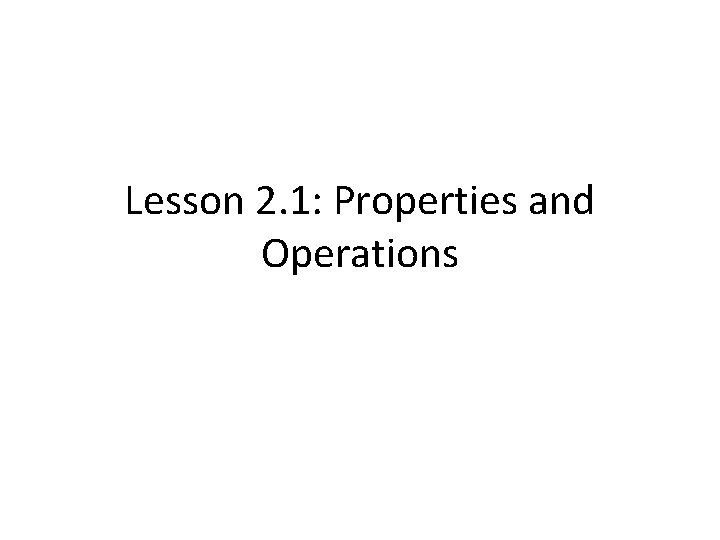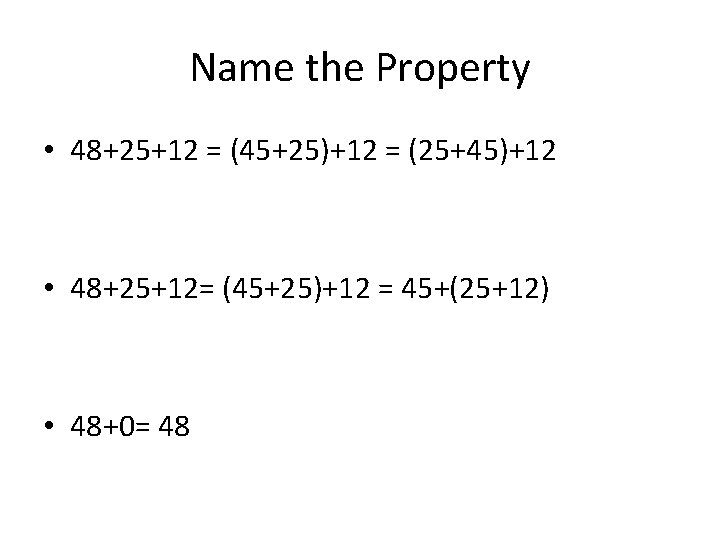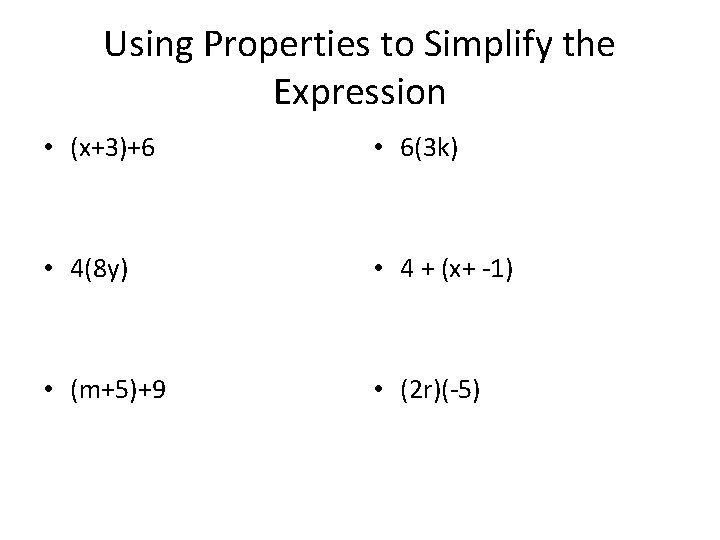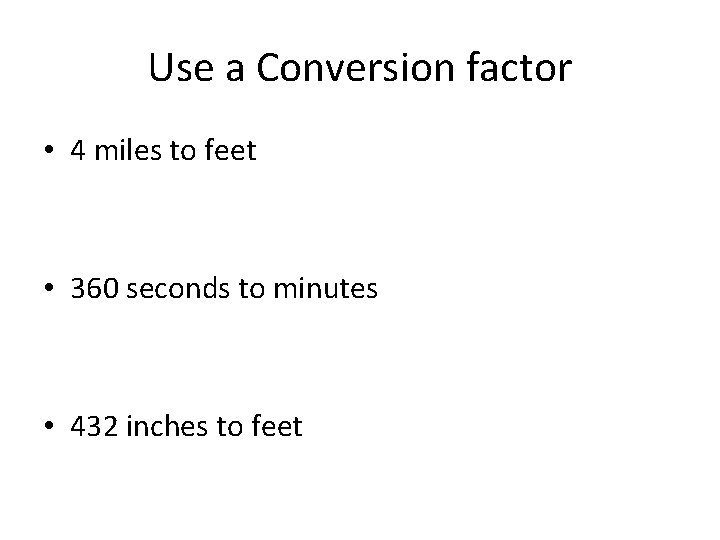# Lesson 2 1 Properties and Operations Commutative Property

• Slides: 10
Download presentationLesson 2. 1: Properties and OperationsCommutative Property • In the sum you can add the numbers in any order. • a+b = b+a • In the product you can multiply the numbers in any order • ab= baAssociative Property • Changing the grouping of numbers in a sum does not change the sum • (a+b)+c = (b+c)+a • Changing the grouping of the numbers in a product does not change the product • (ab)c= a(bc)Identity Properties • The sum of a number and the additive identity (0) is the number • a+0=a • The product of a number and the multiplicative inverse (1) is the number • a· 1 = aName the Property • 48+25+12 = (45+25)+12 = (25+45)+12 • 48+25+12= (45+25)+12 = 45+(25+12) • 48+0= 48Name the Property • (5· 4)· 3 = (4· 5)· 3 • (5· 3)· 4 = (5· 4)· 3 • (-5)(1) = -5Using Properties to Simplify the Expression • (x+3)+6 • 6(3 k) • 4(8 y) • 4 + (x+ -1) • (m+5)+9 • (2 r)(-5)Multiplying by a Conversion Factor • The Steel Dragon 2000 is the world’s longest roller coaster. Its length is 2711 yards. How long is the roller coaster in feet?Evaluate the Expression • 15(-9)(2) • x+17+12 • 7· 1+0 • -8(6 c) • 45+29+55 • 5· 9· (-4)Use a Conversion factor • 4 miles to feet • 360 seconds to minutes • 432 inches to feet